Function Repository Resource:

# LahL

Evaluate the Lah number

Contributed by: Jan Mangaldan
 ResourceFunction["LahL"][n,m] gives the Lah number L(n,m).

## Details

Integer mathematical function, suitable for both symbolic and numerical manipulation.
L(n,m) gives the number of ways of partitioning a set of n elements into m non-empty linearly ordered subsets.

## Examples

### Basic Examples (1)

Evaluate some Lah numbers:

 In:=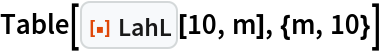Out=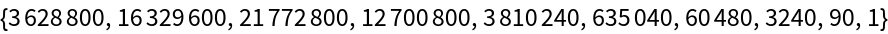### Scope (1)

 In:=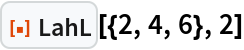Out=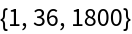### Applications (3)

Plot Lah numbers on a logarithmic scale:

 In:=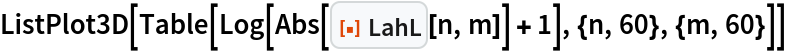Out=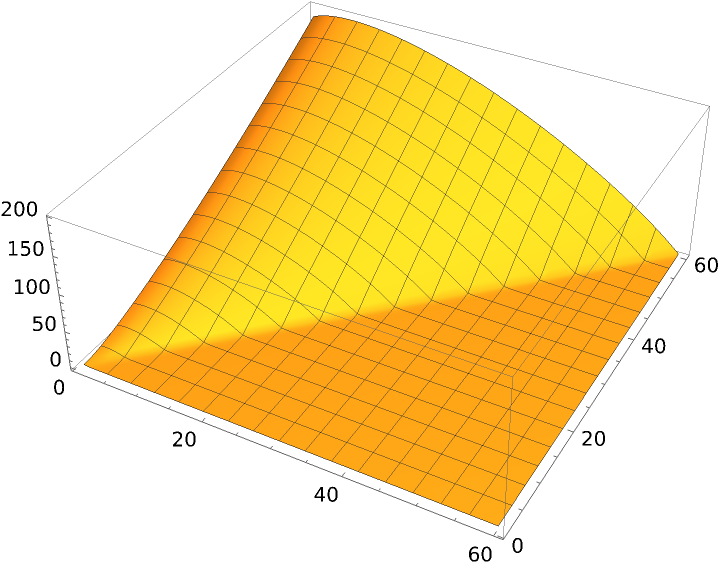Express Pochhammer as a linear combination of FactorialPower:

 In:=Out=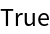Express FactorialPower as a linear combination of Pochhammer:

 In:=Out=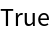Closed form of derivatives of 1/x:

 In:=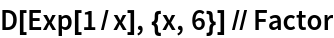Out=In:=Out=### Properties and Relations (3)

Generate values from the generating function:

 In:=Out=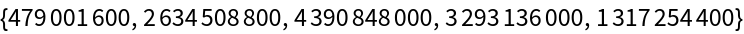In:=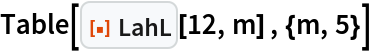Out=Lah numbers can be expressed in terms of Stirling numbers of both kinds:

 In:=Out=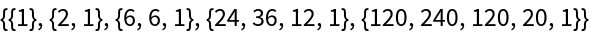In:=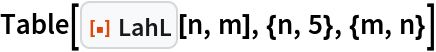Out=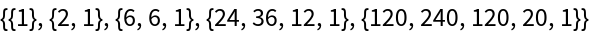Lah numbers are given by a partial Bell polynomial with factorial arguments:

 In:=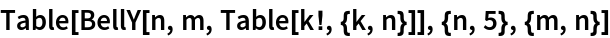Out=In:=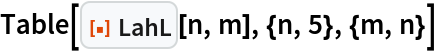Out=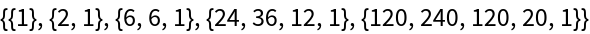## Version History

• 1.0.0 – 17 May 2021